International
Tables for
Crystallography
Volume A
Space-group symmetry
Edited by M. I. Aroyo

International Tables for Crystallography (2016). Vol. A, ch. 3.6, pp. 857-863

## Section 3.6.3. Tables of properties of magnetic groups

D. B. Litvina*

aDepartment of Physics, The Eberly College of Science, Penn State – Berks Campus, The Pennsylvania State University, PO Box 7009, Reading, PA 19610–6009, USA
Correspondence e-mail: u3c@psu.edu

### 3.6.3. Tables of properties of magnetic groups

| top | pdf |

In this section we present a guide to the tables of properties of the two- and three-dimensional magnetic subperiodic groups and the one-, two- and three-dimensional magnetic space groups given by Litvin (2013). The format and content of these magnetic group tables are similar to the format and content of the space-group tables in the present volume, the subperiodic group tables in IT E, and previous compilations of magnetic subperiodic groups (Litvin, 2005) and magnetic space groups (Litvin, 2008b). An example of one these tables is given in Fig. 3.6.3.1. The tables of properties of magnetic groups contain:Figure 3.6.3.1 | top | pdf |Table of properties of the three-dimensional magnetic space group 51.13.399 P2bm′ma.

First page:

(1) Lattice diagram

(3) Diagrams of symmetry elements and of the general positions

(4) Origin

(5) Asymmetric unit

(6) Symmetry operations

Subsequent pages:

(8) Generators selected

(9) General and special positions with spins (magnetic moments)

(10) Symmetry of special projections

Tabulations of properties of three-dimensional magnetic space groups can also be found in Koptsik (1968) (note that the general-position diagrams are of black and white' objects, not spins). Neutron-diffraction extinctions can be found in the work of Ozerov (1969a,b) and on the Bilbao Crystallographic Server, http://www.cryst.ehu.es (Aroyo et al., 2006). General positions and Wyckoff positions of the three-dimensional magnetic space groups can also be found on the Bilbao Crystallographic Server.

#### 3.6.3.1. Lattice diagram

| top | pdf |

For each three-dimensional magnetic space group, a three-dimensional lattice diagram is given in the upper left-hand corner of the first page of the tables of properties of that group. (For all other magnetic groups, the corresponding lattice diagram is given within the symmetry diagram, see Section 3.6.3.3below.) This lattice diagram depicts the coordinate system used, the conventional unit cell of the space group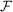, the magnetic space group's magnetic superfamily type and the generators of the translational subgroup of the magnetic space group. In Fig. 3.6.3.2 we show lattice diagrams for two orthorhombic magnetic space groups: (a) Pmc21 and (b) P2bmc′21. The generating lattice vectors are colour coded. Those coloured black are not coupled with time inversion, while those coloured red are coupled with time inversion. In the group Pmc21, a magnetic group of the type, the lattice is an orthorhombic P lattice, see Fig. 3.6.3.2(a), and no generating translation is coupled with time inversion. In the second group, P2bmc′21, a magnetic group of type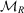, the lattice is an orthorhombic P2b lattice, see Fig. 3.6.3.2(b), and the generating lattice vector in the y direction is coupled with time inversion.Figure 3.6.3.2 | top | pdf |Lattice diagrams of (a) the three-dimensional magnetic space group 26.1.168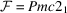and (b) the three-dimensional magnetic space group 26.10.177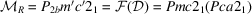.

| top | pdf |

Each table begins with a headline consisting of two lines with five entries, for example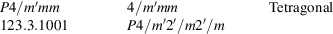For three-dimensional magnetic space groups, this headline is to the right of the lattice diagram. On the upper line, starting on the left, are three entries:

 (1) The short international (Hermann–Mauguin) symbol of the magnetic space group. Each symbol has two meanings: The first is that of the Hermann–Mauguin symbol of a magnetic space-group type. The second is that of a specific magnetic space group, the representative magnetic space group (see Section 3.6.2.2), which belongs to this magnetic space-group type. Given a coordinate system, this group is defined by the list of symmetry operations (see Section 3.6.3.6) given on the page with this Hermann–Mauguin symbol in the heading, or by the given list of general positions and magnetic moments (see Section 3.6.3.9). (2) The short international (Hermann–Mauguin) point group symbol for the geometric crystal class to which the magnetic space group belongs. (3) The crystal system or crystal system/Bravais system classification to which the magnetic space group belongs.

The second line has two additional entries:

 (4) The three-part numerical serial index of the magnetic group (see Section 3.6.2.2.1). (5) The full international (Hermann–Mauguin) symbol of the magnetic space group.

#### 3.6.3.3. Diagrams of symmetry elements and of the general positions

| top | pdf |

There are two types of diagrams: symmetry diagrams and general-position diagrams. The symmetry diagrams show (1) the relative locations and orientations of the symmetry elements and (2) the absolute locations and orientations of these symmetry elements in a given coordinate system. The general-position diagrams show, in that coordinate system, the arrangement of a set of symmetry-equivalent general points and the relative orientations of magnetic moments on this set of points. Figs. 3.6.3.3and 3.6.3.4show the symmetry diagram and general-position diagram, respectively, of the three-dimensional magnetic space group P412′2′.Figure 3.6.3.3 | top | pdf |Symmetry diagram of P412′2′Figure 3.6.3.4 | top | pdf |General-position diagram of P412′2′.

All diagrams of three-dimensional magnetic space groups and three-dimensional subperiodic groups are orthogonal projections. The projection direction is along a basis vector of the conventional crystallographic coordinate system, see Table 1.1 of Litvin (2013). If the other two basis vectors are not parallel to the plane of the diagram, they are indicated by a subscript p, e.g. ap, bp and cp. Schematic representations of the diagrams, showing their conventional coordinate systems, i.e. the origin O and basis vectors, are given in Table 2.1 of Litvin (2013). For two-dimensional magnetic space groups and magnetic frieze groups, the diagrams are in the plane defined by the group's conventional coordinate system.

The graphical symbols used in the symmetry diagrams are listed in Table 2.2 of Litvin (2013) and are an extension of those used in the present volume, IT E and Litvin (2008b). For symmetry planes and symmetry axes parallel to the plane of diagram, for rotation-inversions and for centres of symmetry, the heights' h along the projection direction above the plane of the diagram are given. The heights are given as fractions of the shortest translation along the projection direction and, if different from zero, are printed next to the graphical symbol, see Fig. 3.6.3.3.

In the general-position diagrams, the general positions and corresponding magnetic moments are colour coded. Positions with a z component of +z are shown as red circles and those with a z component of −z are shown as blue circles. If the z component is either h + z or hz with h ≠ 0, then the height h is printed next to the general position, see Fig. 3.6.3.4. If two general positions have the same x component and y component, but one has a z component +z and the other −z, the positions are shown as a circle with one half coloured red, the other half blue. The magnetic moments are colour coded to the general position to which they are associated, their direction in the plane of projection is given by an arrow in the direction of the projected magnetic moment. A + or − sign near the tip of the arrow indicates that the magnetic moment is inclined, respectively, above or below the plane of projection.

For magnetic space groups of the type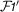, the symmetry diagram is that of the group. That each symmetry element also appears coupled with time inversion is represented by a red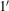printed between and above the general-position and symmetry diagrams. Because groups of this type contain the time-inversion sym­metry, the magnetic moments are all identically zero, and no arrows appear in the general-position diagram. An example, the diagrams of the magnetic space group P41221′ are shown in Fig. 3.6.3.5. For triclinic, monoclinic/oblique, monoclinic/rectangular and orthorhombic rod groups, the colour coding of the general positions is extended according to the positive or negative values of the x and z components of the coordinates of the general position, see Fig. 3.6.3.6.Figure 3.6.3.5 | top | pdf |Diagrams of the magnetic space group P41221′.Figure 3.6.3.6 | top | pdf |General-position diagram of rod group 2.3.29 P2/c′11. The positional colour coding is red for x > 0 and z > 0; blue for x > 0 and z < 0; green for x < 0 and z > 0; and brown for x < 0 and z < 0.

VRML (Virtual Reality Modeling Language) general-position diagrams are available for the two- and three-dimensional magnetic subperiodic groups (Cordisco & Litvin, 2004), and for the one-, two- and non-cubic three-dimensional magnetic space groups (Burke et al., 2006). These diagrams can be rotated and zoomed in on to aid in the visualization of the general-position diagrams, and include both the general positions of the atoms and the general orientations of the associated magnetic moments.

#### 3.6.3.4. Origin

| top | pdf |

If the magnetic space group is centrosymmetric, then the inversion centre or a position of high site symmetry, as on the fourfold axis of tetragonal groups, is chosen as the origin. For noncentrosymmetric groups, the origin is at a point of highest site symmetry. If no site symmetry is higher than 1, the origin is placed on a screw axis, a glide plane or at the intersection of several such symmetries.

In the Origin line below the diagrams, the site symmetry of the origin is given. An additional symbol indicates all symmetry elements that pass through the origin. For example, for the magnetic space group I4/mcm, one finds Origin at center (4/m) at 4/mc21/c'. The site symmetry is 4/m and, in addition, two glide planes perpendicular to the y and z axes, and a screw axis parallel to the z axis, pass through the origin.

#### 3.6.3.5. Asymmetric unit

| top | pdf |

An asymmetric unit is a simply connected smallest part of space which, by application of all symmetry operations of the magnetic group, exactly fills the whole space. For subperiodic groups, because the translational symmetry is of a lower dimension than that of the space, the asymmetric unit is infinite in size. The asymmetric unit for subperiodic groups is defined by setting the limits on the coordinates of points contained in the asymmetric unit. For example, the asymmetric unit for the magnetic layer group 32.3.199 pm′21n′ is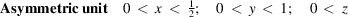Since the translational symmetry of a magnetic space group is of the same dimension as that of the space, the asymmetric unit is a finite part of space. The asymmetric unit is defined, as above, by setting the limits on the coordinates of points contained in the asymmetric unit. For example, for the magnetic space group 140.3.1198 I4/mcm one hasDrawings showing the boundary planes occurring in the tetragonal, trigonal and hexagonal systems, together with their algebraic equations, are given in Fig. 2.1.3.11. Drawings of asymmetric units for cubic groups have been published by Koch & Fischer (1974). The asymmetric units have complicated shapes in the trigonal, hexagonal and cubic crystal systems, and consequently are also specified by giving the vertices of the asymmetric unit. For example, for the magnetic space group 176.1.1374 P63/m one finds

 Asymmetric unit 0 < x < 2/3; 0 < y < 2/3; 0 < z < 1/4; x < (1 + y)/2; y < min(1 − x, (1 + x)/2) Vertices 0, 0, 0 1/2, 0, 0 2/3, 1/3, 0 1/3, 2/3, 0 0, 1/2, 0 0, 0, 1/4 1/2, 0, 1/4 2/3, 1/3, 1/4 1/3, 2/3, 1/4 0, 1/2, 1/4

Because the asymmetric unit is invariant under time inversion, all magnetic space groups,and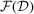of the magnetic superfamily of typehave identical asymmetric units, the asymmetric unit of the group(as in the present volume).

#### 3.6.3.6. Symmetry operations

| top | pdf |

Listed under the heading of Symmetry operations is the geometric description of the symmetry operations of the magnetic group. A symbol denoting the geometric description of each symmetry operation is given. Details of this symbolism, except for the use of prime to denote time inversion, are given in Sections 1.4.2and 2.1.3.9. For glide planes and screw axes, the glide and screw part are always explicitly given in parentheses by fractional coordinates, i.e. by fractions of the basis vectors of the coordinate system ofof the superfamily of the magnetic group. A coordinate triplet indicating the location and orientation of the symmetry element is given, and for rotation-inversions the location of the inversion point is also given. These symbols, with the addition of a prime to denote time inversion, follow those used in the present volume, IT E and Litvin (2005, 2008b). In addition, each symmetry operation is also given in Seitz (1934, 1935a,b, 1936) notation (see Section 3.6.2.2.3), e.g. see Table 3.6.3.1for the symmetry operations of the magnetic space group 51.14.400 P2bmma′.

 Table 3.6.3.1| top | pdf | Symmetry operations of magnetic space group 51.14.400 P2bmma′
 Symmetry operations For (0, 0, 0)+ set (1) 1 (2) 2 1/4, 0, z (3) 2′ 0, y, 0 (4) 2′ (1/2, 0, 0) x, 0, 0 {1∣0} {2001∣1/2, 0, 0} {2010∣0}′ {2100∣1/2, 0, 0}′ (5)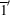(6) a′ (1/2, 0, 0) x, y, 0 (7) m x, 0, z (8) m 1/4, y, z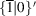{m001∣1/2, 0, 0}′ {m010∣0} {m100∣1/2, 0, 0} For (0, 1, 0)′+ set (1) t′ (0, 1, 0) (2) 2′ 1/4, 1/2, z (3) 2 (0, 1, 0) 0, y, 0 (4) 2 (1/2, 0, 0) x, 1/2, 0 {1∣0, 1, 0}′ {2001∣1/2, 1, 0}′ {2010∣0, 1, 0} {2100∣1/2, 1, 0} (5)0, 1/2, 0 (6) n (1/2, 1, 0) x, y, 0 (7) m′ x, 1/2, z (8) b (0, 1, 0) 1/4, y, z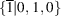{m001∣1/2, 1, 0} {m010∣0, 1, 0}′ {m100∣1/2, 1, 0}′

The corresponding coordinate triplets of the General positions, see Section 3.6.3.9, may be interpreted as a second description of the symmetry operations, a description in matrix form. The numbering (1), (2), …, (p), … of the entries in the blocks Symmetry operations is the same as the numbering of the corresponding coordinate triplets of the General position, the first block below Positions. For all magnetic groups with primitive lattices, the two lists, Symmetry operations and General position, have the same number of entries.

For magnetic groups with centred cells, only one block of several (two, three or four) blocks of the general positions is explicitly given, see Table 3.6.3.2. A set of two, three or four centring translations is given below the subheading Coordinates. Each of these translations is added to the given block of general positions to obtain the complete set of blocks of general positions. While one of the several blocks of general positions is explicitly given, the corresponding symmetry operations are all explicitly given. Each corresponding block of symmetry operations is listed under a subheading of centring translation + set' for each centring translation listed below the subheading Coordinates.

 Table 3.6.3.2| top | pdf | General positions of magnetic space group 51.14.400 P2bmma′
 Positions Coordinates (0, 0, 0)+ (0, 1, 0)′+ 16 l 1 (1) x, y, z [u, v, w] (2)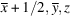[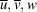] (3)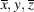[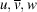] (4)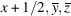[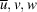] (5)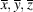[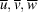] (6)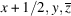[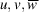] (7)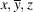[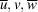] (8)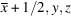[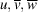]

#### 3.6.3.7. Abbreviated headline

| top | pdf |

On the second and subsequent pages of the tables for a specific magnetic group there is an abbreviated headline. This abbreviated headline contains three items: (1) the word Continued', (2) the three-part number of the magnetic group type, and (3) the short international (Hermann–Mauguin) symbol for the magnetic group type.

#### 3.6.3.8. Generators selected

| top | pdf |

The line Generators selected lists the symmetry operations selected to generate the symmetry-equivalent points of the General position from a point with coordinates x, y, z. The first generator is always the identity operation given by (1) followed by generating translations. Additional generators are given as numbers (p), which refer to the coordinate triplets of the General position and to corresponding symmetry operations in the first block, if more than one, of the Symmetry operations.

#### 3.6.3.9. General and special positions with spins (magnetic moments)

| top | pdf |

The entries under Positions, referred to as Wyckoff positions, consist of the General position, the upper block, followed by blocks of Special positions. The upper block of positions, the general position, is a set of symmetry-equivalent points where each point is left invariant only by the identity operation or, for magnetic groups, by the identity operation and time inversion, but by no other symmetry operations of the magnetic group. The lower blocks, the special positions, are sets of symmetry-equivalent points where each point is left invariant by at least one additional operation in addition to the identity operation, or, for magnetic space groups, in addition to the identity operation and time inversion.

For each block of positions the following information is provided:

Multiplicity: The multiplicity is the number of equivalent positions in the conventional unit cell of the non-primed groupassociated with the magnetic group.

Wyckoff letter: This letter is a coding scheme for the blocks of positions, starting with a' at the bottom block and continuing upwards in alphabetical order.

Site symmetry: The site-symmetry group is the largest subgroup of the magnetic space group that leaves invariant the first position in each block of positions. This group is isomorphic to a subgroup of the point group of the magnetic group. An oriented' symbol is used to show how the symmetry elements at a site are related to the conventional crystallographic basis, and the sequence of characters in the symbol correspond to the sequence of symmetry directions in the magnetic group symbol. Sets of equivalent symmetry directions that do not contribute any element to the site symmetry are represented by dots. Sets of symmetry directions having more than one equivalent direction may require more than one character if the site-symmetry group belongs to a lower crystal system. For example, for the 2c position of the magnetic space group P4′mm (99.3.825) the site-symmetry group is 2mm′.'. The two characters mm′ represent the secondary set of tetragonal symmetry directions, whereas the dot represents the tertiary tetragonal symmetry directions.

Coordinates of positions and components of magnetic moments: In each block of positions, the coordinates of each position are given. Immediately following each set of position coordinates are the components of the symmetry-allowed magnetic moment at that position. The components of the magnetic moment of the first position are determined from the given site-symmetry group. The components of the magnetic moments at the remaining positions are determined by applying the symmetry operations to the components of that magnetic moment at the first position.

#### 3.6.3.10. Symmetry of special projections

| top | pdf |

The symmetry of special projections is given for the two- and three-dimensional magnetic groups. For each three-dimensional magnetic group, the symmetry is given for three projections, projections onto planes normal to the projection directions. If there are three symmetry directions, the three projection directions correspond to primary, secondary and tertiary symmetry directions. If there are fewer than three symmetry directions, the additional projection direction or directions are taken along coordinate axes. For two-dimensional magnetic groups, there are two orthogonal projections. The projections are onto lines normal to the projection directions.

For the three-dimensional magnetic space groups, each projection gives rise to a two-dimensional magnetic space group. For two-dimensional magnetic space groups, each projection gives rise to a one-dimensional magnetic space group. For magnetic rod groups and magnetic layer groups, a projection along the  direction gives rise, respectively, to a two-dimensional magnetic point group and a two-dimensional magnetic space group. All other projections give rise to magnetic frieze groups. For magnetic frieze groups, projections give rise to either a one-dimensional magnetic space group or a one-dimensional magnetic point group. The international (Hermann–Mauguin) symbol of the symmetry group of each projection is given. Below this symbol, the basis vector(s) of the projected symmetry group and the origin of the projected symmetry group are given in terms of the basis vector(s) of the projected magnetic group. The location of the origin of the symmetry group of the projection is given with respect to the unit cell of the magnetic group from which it has been projected.

### References

Aroyo, M. I., Perez-Mato, J. M., Capillas, C., Kroumova, E., Ivantchev, S., Madariaga, G., Kirov, A. & Wondratschek, H. (2006). Bilbao Crystallographic Server I: Databases and crystallographic computing programs. Z. Kristallogr. 221, 15–27.
Burke, J. S., Cordisco, N. R. & Litvin, D. B. (2006). VRML general position diagrams of magnetic space groups. J. Appl. Cryst. 39, 620.
Cordisco, N. R. & Litvin, D. B. (2004). VRML general position diagrams of the magnetic subperiodic groups. J. Appl. Cryst. 37, 346.
Koch, E. & Fischer, W. (1974). Zur Bestimmung asymmetrischer Einheiten kubischer Raumgruppen mit Hilfe von Wirkungsbereichen. Acta Cryst. A30, 490–496.
Koptsik, V. A. (1968). A general sketch of the development of the theory of symmetry and its applications in physical crystallography over the past 50 years. Sov. Phys. Crystallogr. 12, 667–683.
Litvin, D. B. (2005). Tables of properties of magnetic subperiodic groups. Acta Cryst. A61, 382–385.
Litvin, D. B. (2008b). Tables of crystallographic properties of magnetic space groups. Acta Cryst. A64, 419–424.
Litvin, D. B. (2013). Magnetic Group Tables, 1-, 2- and 3-Dimensional Magnetic Subperiodic Groups and Space Groups. Chester: International Union of Crystallography. Freely available from http://www.iucr.org/publ/978–0–9553602–2–0 .
Ozerov, R. P. (1969a). The design of tables of Shubnikov space groups of dichroic symmetry. Kristallografiya, 14, 393–403.
Ozerov, R. P. (1969b). The design of tables of Shubnikov space groups of dichroic symmetry. Sov. Phys. Crystallogr. 14, 323–332.
Seitz, F. Z. (1934). A matrix-algebraic development of the crystallographic groups. Z. Kristallogr. 88, 433–459.
Seitz, F. Z. (1935a). A matrix-algebraic development of the crystallographic groups. Z. Kristallogr. 90, 289–313.
Seitz, F. Z. (1935b). A matrix-algebraic development of the crystallographic groups. Z. Kristallogr. 91, 336–366.
Seitz, F. Z. (1936). A matrix-algebraic development of the crystallographic groups. Z. Kristallogr. 94, 100–130.